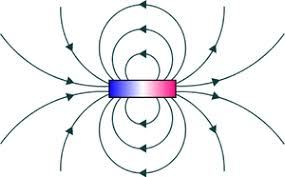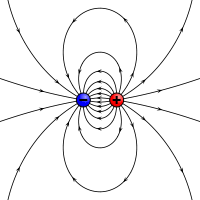## How to Calculate and Solve for Magnetic Potential | Magnetic FieldThe image above represents magnetic potential.

To compute for magnetic potential, four essential parameters are needed and these parameters are Magnetic Permeability of Vacuum (μo), Strength of Magnetic Pole (m), Relative Magnetic Permeability (μRand Seperating Distance (r).

The formula for calculating magnetic potential:

v = μom / 4πμRr

Where:

v = Magnetic Potential
μo = Magnetic Permeability of Vacuum
m = Strength of Magnetic Pole
μR = Relative Magnetic Permeability
r = Separating Distance

Let’s solve an example;
Find the magnetic potential when the magnetic permeability of vaccum is 1.2566371E-6, the strength of magnetic pole is 10, the relative magnetic permeability is 22 and seperating distance is 18.

This implies that;

μo = Magnetic Permeability of Vacuum = 1.2566371E-6
m = Strength of Magnetic Pole = 10
μR = Relative Magnetic Permeability = 22
r = Separating Distance = 18

v = μom / 4πμRr
v = (0.0000012566371)(10) / 4π(22)(18)
v = 0.000012566371000000001 / 4976.282
v = 2.52e-9

Therefore, the magnetic potential is 2.52e-9.

## How to Calculate and Solve for Magnetic Field | Magnetic FieldThe image above represents magnetic field.

To compute for magnetic field, four essential parameters are needed and these parameters are Magnetic Permeability of Vacuum (μo), Strength of Magnetic Pole (m), Relative Magnetic Permeability (μRand Seperating Distance (r).

The formula for calculating magentic field:

B = μom / 4πμRr²

Where:

B = Magnetic Field
μo = Magnetic Permeability of Vacuum
m = Strength of Magnetic Pole
μR = Relative Magnetic Permeability
r = Separating Distance

Let’s solve an example;
Find the magnetic field when the magnetic permeability of vaccum is 1.2566371E-6, strength of magnetic pole is 11, relative magnetic permeability is 9 and the seperating distance is 12.

This implies that;

μo = Magnetic Permeability of Vacuum = 1.2566371E-6
m = Strength of Magnetic Pole = 11
μR = Relative Magnetic Permeability = 9
r = Separating Distance = 12

B = μom / 4πμRr²
B = (0.0000012566371)(11) / 4π(9)(12)²
B = 0.00001382 / 4π(9)(144)
B = 0.00001382 / (16286.0163)
B = 8.487e-10

Therefore, the magnetic field is 8.487e-10.